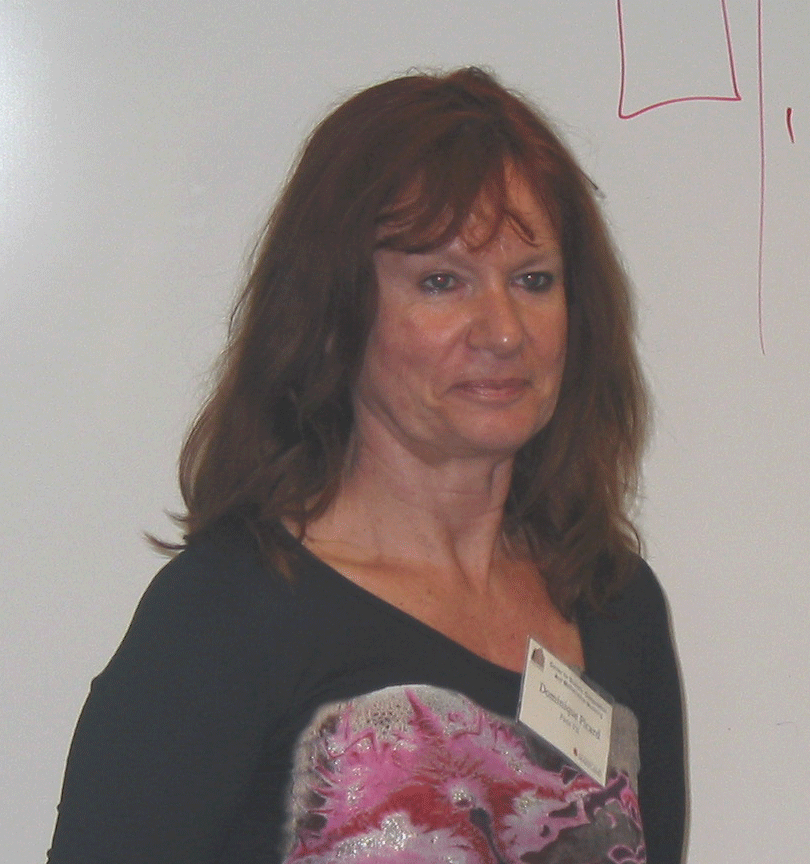[ Search | Site Map | Contact ]Research Activities > Programs > Sparse Representation in Redundant Systems > Dominique Picard

##### Sparse Representation in Redundant SystemsCSIC Building (#406), Seminar Room 4122.
Directions: home.cscamm.umd.edu/directions### Functional Estimation for Inverse Problems

Dr. Dominique Picard

Statistics at Paris VII

Abstract:   We consider the usual formulation of a statistical linear inverse problem : an unknown object of interest f is to be recovered from y" = Kf + " ˙W where y" is the observation, K is a known linear operator, and ˙W is a gaussian white noise model. The most typical example corresponds to the case where K is the convolution with a regular and known function . - or, which is equivalent, to estimate the density probability of a random variable X, when we observe Z1, . . . ,Zn, iid, Zi = Xi +Ui, the Ui's are independent of the Xi's and with a fixed law.

The main difficulty lies in the fact that this problem contains two kind of bases, which are natural but may be antagonistic : the singular value decomposition basis (SVD), which allows explicit calculation and preserve the decorrelation structure of the noise (in the convolution case, it is the Fourier basis) and a basis (typically a WAVELET basis) in which one can easily express the regularity and perform Lp calculations. We provide here a method (WAVE-VD) taking advantage of both bases and allowing to directly estimate and threshold the wavelet coefficients. We show that this method achieve minimax rates of convergence in a large class of spaces, depending on the regularity of the operator K. We investigate the conditions on the operator allowing to well describe the rate of convergence of the method. We show that these conditions express in terms of sparsity of the operator, and that in the convolution case with a box-car function (I{x 2 [a, a + 1]}) these conditions express in terms of the diophantian properties of the real number a. We show that this method also allows to consider random operator K  and even partially observed operator.UM Home | Directories | Search | Admissions | Calendar | Web Accessibility Maintained by CSCAMM Direct questions and comments to webmaster@cscamm.umd.edu CSCAMM is part of the College of Computer, Mathematical & Natural Sciences (CMNS)